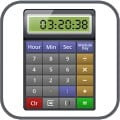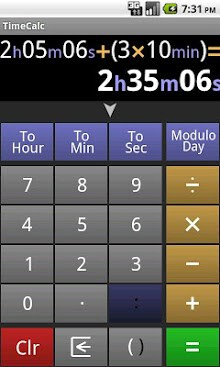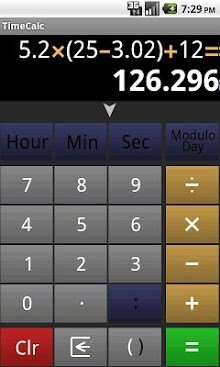# Time Calculator APKTimeCalc is a calculator to operate on time and numbers.
The calculator supports parentheses and the operator priority.
You can copy/paste results from the calculator to other android applications.
You can see the history of all operations done with the calculator during the current session.
You can add, subtract, divide times between them.
2h 20m 3s – 1h 20m = 1h 00m 03s
30 min / 10 min = 3

You can do operations on numbers like on a regular calculator.
2.5 + 3 * 5 = 17.5

You can do operations between times and numbers.
2 * 25 min = 50 min
1 hr / 2 = 30 min

You can enter time as integer with the format xx Hr xx Min xx Sec, or as floating numbers.
8h 25m 13s
8:25:13
3.5 hr

The calculator supports 12h (AM/PM) or 24h time format.
8:00:00 PM
20:00:00

You can convert times to another unit.
2 hr 10 min 3 sec = 2.168 hr = 130.05 min = 7803 sec

You can do ‘modulo day’ to convert a time to a day time.
6:00:00 PM + 14 hr = 32 hr
32 hr Modulo 24h = 8:00:00 AM

The calculator supports parentheses and the operator priority (multiplication and division have higher priority than addition and subtraction).
(2 + 3) * (20 – 2 * 10) = 5 * 1 = 5

Size : 117k
Current Version : 1.05
Requires Android : 2.2 and up
Offered By : AndrololoidThis site uses Akismet to reduce spam. Learn how your comment data is processed.